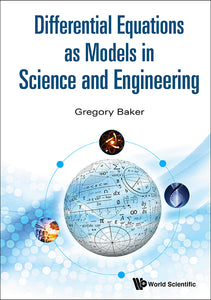# Differential Equations As Models In Science And Engineering

Gregory Richard Baker

Format: Print Book

ISBN: 9789814656962

• SGD 131.61
Unit price per
Tax included.

This textbook develops a coherent view of differential equations by progressing through a series of typical examples in science and engineering that arise as mathematical models. All steps of the modeling process are covered: formulation of a mathematical model; the development and use of mathematical concepts that lead to constructive solutions; validation of the solutions; and consideration of the consequences. The volume engages students in thinking mathematically, while emphasizing the power and relevance of mathematics in science and engineering. There are just a few guidelines that bring coherence to the construction of solutions as the book progresses through ordinary to partial differential equations using examples from mixing, electric circuits, chemical reactions and transport processes, among others. The development of differential equations as mathematical models and the construction of their solution is placed center stage in this volume.

Format: Hardcover
No of Pages: 392
Imprint: World Scientific
Publication date: 20160726